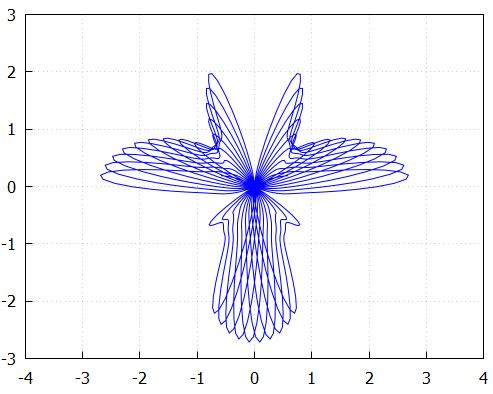• >
• >

试用计算软件计算下列函数的图形：

(4)

实验代码：

r(t):=sin(2.9*t)*%e^(sin(4.9*t))^4;
wxdraw2d(proportional_axes=xy,
grid=true,xtics=[-5,1,5],ytics=[-5,1,5],nticks=1200,
xrange=[-4,4],yrange=[-3,3],
polar(r(t),t,-4.9*%pi,4.9*%pi)
);

执行结果